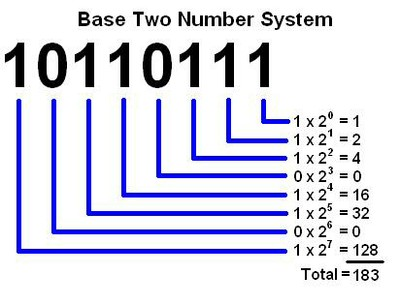# Zachary Lopez

## Bitwise Operations in Javascript

Bitwise Operations are a way of manipulating data at the most basic level of individual bits. This introduces some complexity as the data representation isn’t as clear, but the tradeoff is a massive gain in speed. The speed gain comes because the operations are native to the processor and are some of the most basic commands that a computer must be able to execute.

Over simple applications or programs this may not mean much, however, when your program is computationally heavy and speed is a factor this approach will save cpu time. An example of this is the work that Andy Coenen and I did on the NQueens project.

Switching to bitwise operations allowed us to reach a higher and higher number N on a single computer.

In this post, I will cover what the basic bitwise operations are and how to use them.

## Background

The first thing to understand is how numbers are represented in binary. Our number system is base 10, so each digit represents a number that is then multiplied by 10 to the power of position of the digit. Ugh, that is not so clear. Let’s try to diagram that a bit.In a binary, or base 2, system each place can either be 0 or 1 and is multiplied by 2 to the power of position. Again an image.Using binary, you can represent numbers, but the power comes when you can represent other values. A good example is true or false, where true takes the value of 1, and false takes the value of 0.

The following are some of the opations you can use.

Addition can be achieved by bit shifting to the left.

``````var value = 1;
value = value<<1;
console.log(value) //2
console.log(value.toString(2)); //10

``````

Notice that you can use the toString function with a value of 2 to indicate base to to get the binary representation back. This applies for all of the functions and will be useful as you work with bitwise operations.

## Subtraction (right bitshift)

Subtraction can be achieved by doing the opposite of addition and shifting bits to the right.

``````var value = 2;
value = value>>1;
console.log(value) //1
console.log(value.toString(2)); //01

``````

## Bitwise AND

This function (&) returns a 1 in each bit position where a 1 exists in both bit positions of the values being compared.

``````var a = 3;
a.toString(2); //11
var b = 1;
b.toString(2); //01

var result = a & b;
result.toString(2); //01
``````

## Bitwise OR

 This function ( )returns a 1 in each bit position where a 1 exists in either bit position of the values being compared.
``````var a = 3;
a.toString(2); //11
var b = 1;
b.toString(2); //01

var result = a | b;
result.toString(2); //11
``````

## Bitwise XOR

This function (^) returns a 1 in each bit position where a 1 exists in one bit position, but not the other, of the values being compared.

``````var a = 3;
a.toString(2); //11
var b = 1;
b.toString(2); //01

var result = a | b;
result.toString(2); //10
``````

## Bitwise NOT

This function (~) inverts the bits on a given value.

``````var a = 6;  //110
var b = ~a; //-111 or -7
b.toString(2);
``````

``````var a = 11;  //1011